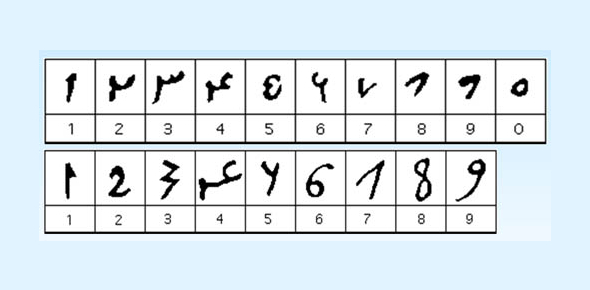# Math Quiz: Sets And The History Of The Numerical System

10 Questions | Total Attempts: 343SettingsThe numerical system has been used for ages since I started in the Sumerian civilization and is the centerpiece of mathematics. The following quiz is about sets and the history of our numeration system. Take it up and get to see just how much you know about this system from your math history lesson. All the best!

• 1.
The numeration system we use today is from_____ and ______ systems.
• A.

China and Japan

• B.

Egypt and Africia

• C.

Hindu and Arabic

• 2.
The seven basic symbols used by the Romans are ________ .
• A.

I, II, IV, X, VI, XI, III.

• B.

I, V, X, L, C, D, M.

• C.

A, O, III, X, IV, VI, I.

• 3.
Our number system is called the ______ system.
• A.

Positional

• B.

Decimal

• C.

Numerical

• 4.
A collection of objects called elements are ________.
• A.

Sets

• B.

Equal

• C.

Union

• 5.
Circles rectangles and other shapes used to illustrate sets are called ________.
• A.

Infinite

• B.

Universal set

• C.

Venn Diagram

• 6.
When it is possible to match each element in one set to exactly one element in the other it is called a ___________.
• A.

Disjoint Sets

• B.

One-to one correspondence

• C.

Finite

• 7.
Sets with nothing in common are called_________.
• A.

Finite

• B.

Universal set

• C.

Disjoint Sets

• 8.
The set that contains all the elements being considered in a situation is called _________.
• A.

Venn diagram

• B.

Union

• C.

Universal set

• 9.
A set that is empty or one that has a definite end is called a _______.
• A.

Infinite

• B.

Equal

• C.

Finite

• 10.
Sets that have the same elements or is a subset of the other is called ________.
• A.

Equal

• B.

Union

• C.

Disjoint set

Related TopicsBack to top